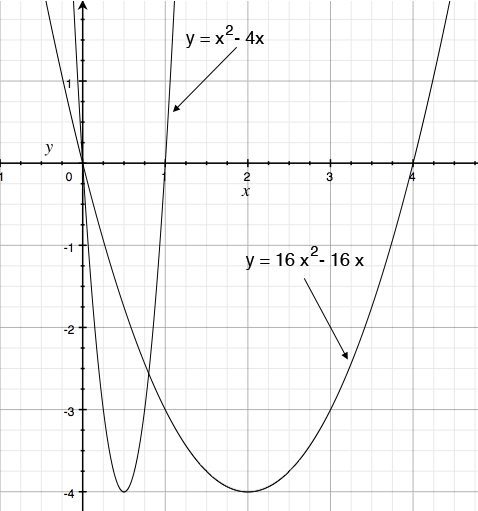SEARCH HOMEMath Central Quandaries & QueriesQuestion from corinanna, a student: Hi, How do you find a quadratic equation given the domain & range, & or the y-intercept?Hi,

There is not enough information to completely determine the quadratic equation. For example I looked at $y = x^2 - 4x$ and $y = 16 x^2 - 16 x.$ For each of them the domain is the real line, the range is all $y \ge -2$ and they each have $y = 0$ as the y-intercept.

I plotted them below.PennyMath Central is supported by the University of Regina and The Pacific Institute for the Mathematical Sciences.